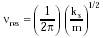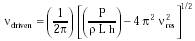Nanomedicine, Volume I: Basic Capabilities

Robert A. Freitas Jr., Nanomedicine, Volume I: Basic Capabilities, Landes Bioscience, Georgetown, TX, 1999

Driving the sensor at a frequency near nres may cause large amplitude excursions unless the motion is heavily damped. The lowest possible undamped resonance frequency nres for piston-type acoustic sensors of size ~ L with minimum spring constant ks ~ 2 kT / L2 for a maximally sensitive spring having an energy of ~kT when stretched by L, and piston mass m, is{Eqn. 4.32}

where m = r L2 h for a piston of area L2, height h, and density r. Assuming h/L = 0.1 and r ~ 1000 kg/m3, nres = 21 MHz for an L = 22 nm sensor (ks = 1.8 x 10-5 N/m) and nres = 4 KHz for an L = 680 nm sensor (ks = 1.9 x 10-8 N/m). Resonance frequency may be adjusted by selecting appropriate ks and m. In practical devices resonance will occur at a slightly lower frequency than nres and with some broadening of the peak, because m should also include the masses of the shank of the piston plus a time-varying portion of the fluid mass occupying the piston's chamber (depending upon the time-varying position of the piston).

Consider the surface of a piston of height h, area L2 and mass m to which a pressure spike of amplitude P (N/m2) and frequency ndriven is applied, producing a stroke length Xstroke. The solution for the equation of motion of an undamped forced harmonic oscillator has a maximum amplitude of Xstroke = Fspike / abs ( m (wdriven2 wres2)) where w = 2 p n, Fspike = P L2 and m is defined above. The driving frequency that produces stroke length Xstroke = L is given by:{Eqn. 4.33}

For an L = 22 nm sensor driven to full throw by a minimum detectable DPmin = 0.03 atm pressure spike (again taking h/L = 0.1 and r ~ 1000 kg/m3), ndriven = 34 MHz; ndriven = 45 GHz when the sensor is driven to full throw by the maximum possible pressure spike Pmax = 39,000 atm consistent with water remaining in the liquid state (see below). The piston may be driven at these frequencies, or slower, using triangular-wave pulse trains of pressure spikes of these magnitudes.

For an L = 680 nm sensor driven to full throw by a minimum detectable DPmin = 10-6 atm pressure spike, ndriven = 6 KHz; ndriven = 1.5 GHz when the sensor is driven to full throw by the maximum pressure spike Pmax = 39,000 atm.

What is the maximum tolerable pressure spike in aqueous media? The requirement of subsonic piston motion in water gives the highest maximum: Pmax < (1/2) r vsound2 (N/m2), if r = 3510 kg/m3 for a solid diamondoid piston and the speed of sound vs ~ 1450 m/sec in water (Eqn. 4.30) for bulk modulus Bwater = 2.2 x 109 N/m2, rwater = 1000 kg/m3, giving Pmax < 39,000 atm. However, rapid dissipative pressure spikes Pmax > 26,000 atm add sufficient energy to boil 310 K water, and isothermal static compression of pure water at 310 K causes crystallization into Ice VI near ~11,500 atm. Additional restrictions on (and features of) high-frequency pressure transducers are described in Sections 6.3.3, 6.4.1, and 7.2.2.

Last updated on 17 February 2003